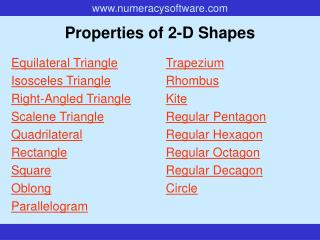DownloadDownload PresentationProperties of 2-D Shapes

# Properties of 2-D Shapes

Download Presentation## Properties of 2-D Shapes

- - - - - - - - - - - - - - - - - - - - - - - - - - - E N D - - - - - - - - - - - - - - - - - - - - - - - - - - -
##### Presentation Transcript

1. Properties of 2-D Shapes Trapezium Rhombus Kite Regular Pentagon Regular Hexagon Regular Octagon Regular Decagon Circle Equilateral Triangle Isosceles Triangle Right-Angled Triangle Scalene Triangle Quadrilateral Rectangle Square Oblong Parallelogram

2. Equilateral Triangle Key Features All three sides are the same lengthand all three angles are equal. Lines of Symmetry? Three. Rotational Symmetry? Order 3.

3. Isosceles Triangle Key Features A triangle with two equal sides and two equal angles. Lines of Symmetry? One. Rotational Symmetry? None.

4. Scalene Triangle Key Features A triangle with three different sides and three different angles. Lines of Symmetry? None. Rotational Symmetry? None.

5. Right-Angled Triangle Key Features A triangle with one right-angle. Can a Triangle be Right-Angled and Isosceles? Yes. Lines of Symmetry? Only if it’s an isosceles triangle - one line. Rotational Symmetry? None.

6. Quadrilateral Key Features A shape with four sides. Lines of Symmetry? It depends on what sort of quadrilateral it is. Rotational Symmetry? It depends on what sort of quadrilateral it is. Names of Special Quadrilaterals Rectangle, Square, Parallelogram, Trapezium, Rhombus, Kite.

7. Rectangle Key Features A quadrilateral with four right-angles.Opposite sides are the same length. Lines of Symmetry? Two, unless the rectangle is a square. Rotational Symmetry? Order 2, unless the rectangle is a square. Is a Rectangle a Parallelogram? Yes, because both pairs of opposite sides are parallel.

8. Square Key Features A quadrilateral with four equal sides and four right-angles. Lines of Symmetry? Four. Rotational Symmetry? Order 4. Is a Square a Rectangle? Yes, because it’s a quadrilateral with four right-angles. Is a Square a Parallelogram? Yes, because both pairs of opposite sides are parallel. Is a Square a Rhombus? Yes, because it’s got four sides all the same length.

9. Parallelogram Key Features A quadrilateral with opposite sides that are parallel and the same length. Lines of Symmetry? Only if it’s a rectangle, a square or a rhombus. Rotational Symmetry? Order 2.

10. Oblong Key Features A rectangle which is not a square. Lines of Symmetry? Two. Rotational Symmetry? Order 2. Is an Oblong a Parallelogram? Yes, because both pairs of opposite sides are parallel.

11. Trapezium Key Features A quadrilateral with just one pair of parallel sides. Lines of Symmetry? Only if it’s an isosceles trapezium. Rotational Symmetry? None.

12. Rhombus Key Features A quadrilateral with four equal sides. Lines of Symmetry? Two. Rotational Symmetry? Order 2. Is a Rhombus a Parallelogram? Yes, because both pairs of opposite sides are parallel.

13. Kite Key Features A quadrilateral with two pairs of adjacent sides that are equal. Lines of Symmetry? One. Rotational Symmetry? None.

14. Regular Pentagon Key Features A shape with five equal sides and five equal angles. Lines of Symmetry? Five. Rotational Symmetry? Order 5.

15. Regular Hexagon Key Features A shape with six equal sides and six equal angles. Lines of Symmetry? Six. Rotational Symmetry? Order 6.

16. Regular Octagon Key Features A shape with eight equal sides and eight equal angles. Lines of Symmetry? Eight. Rotational Symmetry? Order 8.

17. Regular Decagon Key Features A shape with ten equal sides and ten equal angles. Lines of Symmetry? Ten. Rotational Symmetry? Order 10.

18. Circle Key Features Every point on the circumference is the same distance from the centre. Lines of Symmetry? Infinite. Rotational Symmetry? Order infinity.

19. END OF PRESENTATION